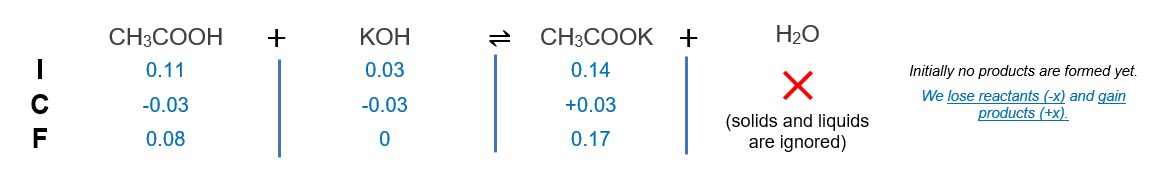# Problem: A buffer solution contains 0.11 mol of acetic acid and 0.14 mol of sodium acetate in 1.00 L.What is the pH of the buffer after the addition of 0.03 mol of KOH?

###### FREE Expert Solution

We are asked to calculate the pH of the buffer after the addition of 0.03 mol of KOH?

Step 1. ICF chartStep 2. Use buffer equation###### Problem Details

A buffer solution contains 0.11 mol of acetic acid and 0.14 mol of sodium acetate in 1.00 L.

What is the pH of the buffer after the addition of 0.03 mol of KOH?